2015/09/08 21:51

# 什么是快速排序

## 快速排序简介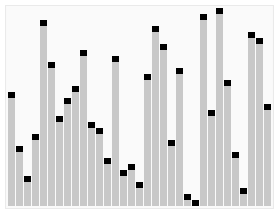## 快速排序的分治思想## 如何划分

Partition(A,p,q)   // A[p,..q]
1   x=A[p]   // pivot=A[p] 主元
2   i=p
3   for j=p+1 to q
4       do if A[j]<=x
5          then i=i+1
6             exch A[i]<->A[j]
7   exch A[p]<->A[i]
8   return i // i pivot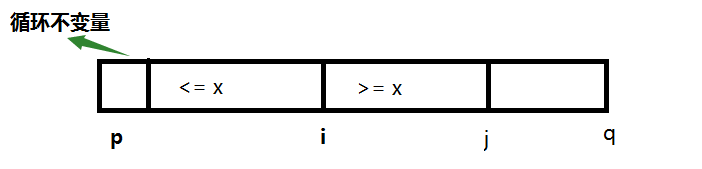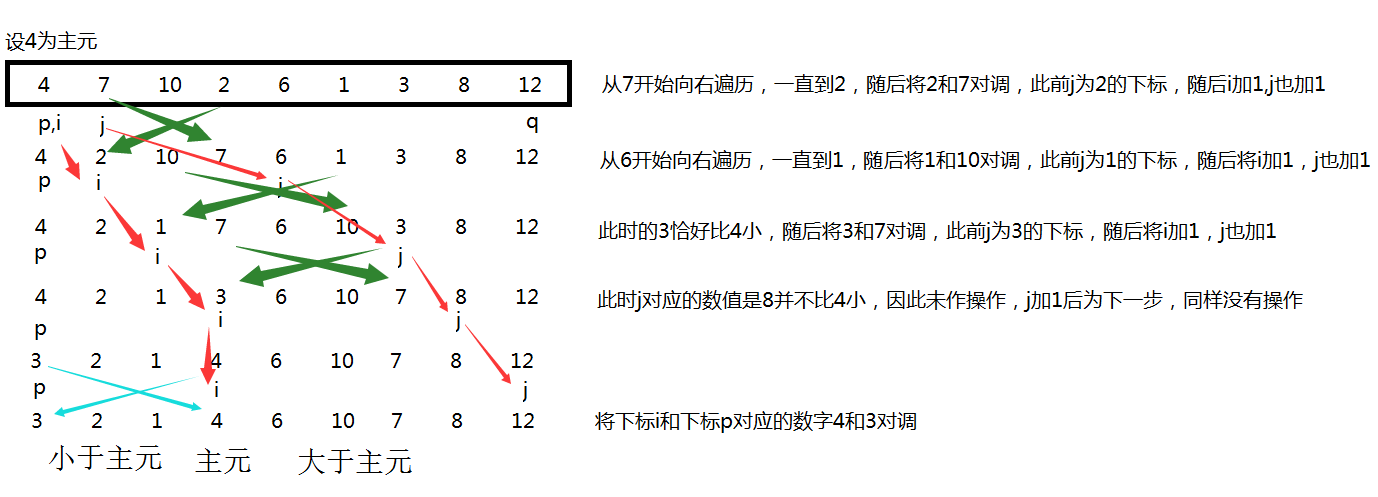## 将递归的思想作用于划分上

Quicksort(A,p,q)
1   if p<q
2     then r=Partition(A,p,q)
3          Quicksort(A,p,r-1)
4          Quicksort(A,r+1,q) 

# 快速排序的算法分析

## 怎样是最坏的划分

1）输入的元素已经排序或逆向排序
2）每个划分的一边都没有元素

T(n)=T(0)+T(n1)+ \Theta(n) =Θ(1)+T(n1)+Θ(n)=Θ(n1)+Θ(n)=Θ(n2)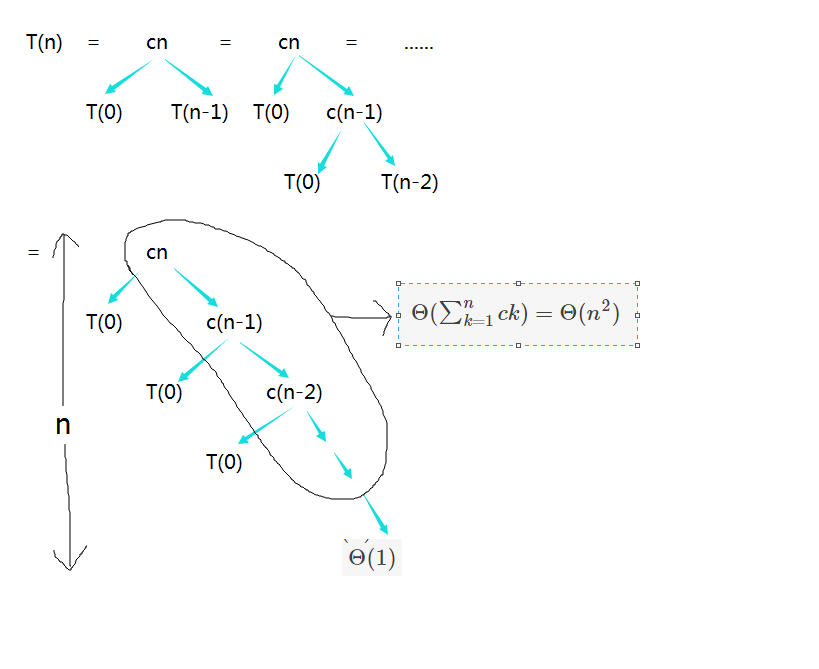T(n)=Θ(n)+Θ(n2)=Θ(n2)

## 最坏划分的算法分析

T(n)=max0rn1(T(r)+T(nr1))+Θ(n)

T(n)max0rn1(cr2+c(nr1)2)+Θ(n)cmax0rn1(r2+(nr1)2)+Θ(n)

1）而 r2+(nr1)2 对于r的二阶导数为正，所以在区间 0rn1 的右端点取得最大值。

T(n)cn2c(2n1)+Θ(n)

2） r2+(nr1)2 对于r的二阶导数为正，所以在区间 0rn1 的左端点取得最小值。

T(n)cn2c(2n1)+Θ(n)

## 怎样是最好的划分

T(n)=2T(n/2)+Θ(n)=Θ(nlgn)

## 怎样是平衡的划分

T(n)=T(110n)+T(910n)+Θ(n)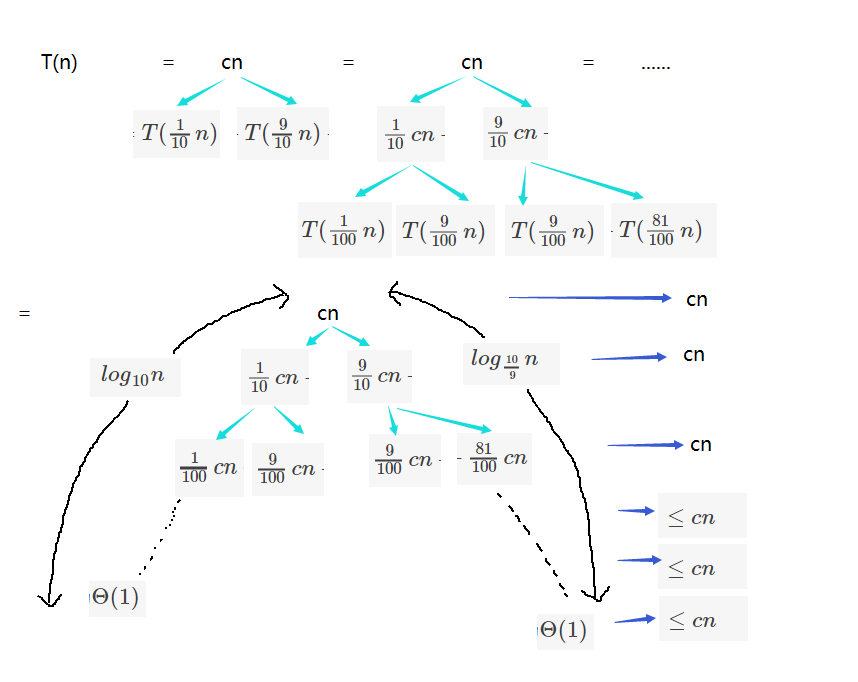T(n)cnlog109n+Θ(n)

T(n)=Θ(nlgn)

# 随机化快速排序

## 随机算法的思想

【算法】3 由招聘问题看随机算法中我们介绍了随机算法，它使得对于所有的输入都有着较好的期望性能，因此随机化快速排序在有大量数据输入的情况下是一种更好的排序算法。

1）其运行时间不依赖与输入序列的顺序

2）无需对输入序列的分布做任何假设

3）没有 一种特别的输入会引起最差的运行情况

4）最差的情况由随机数产生器决定

## 随机抽样技术

RANDOMIZED-PARTITION(A,p,q)
1   i=RANDOM(p,q)
2   exchange A[p] with A[i]
3   return PARTITION(A,p,q)
RANDOMIZED-QUICKSORT(A,p,q)
1   if p<q
2       r=RANDOMIZED-PARTITION(A,p,q)
3       RANDOMIZED-QUICKSORT(A,p,r-1)
4       RANDOMIZED-QUICKSORT(A,r+1,q)

http://blog.csdn.net/nomasp

0
0 收藏

### 作者的其它热门文章0 评论
0 收藏
0